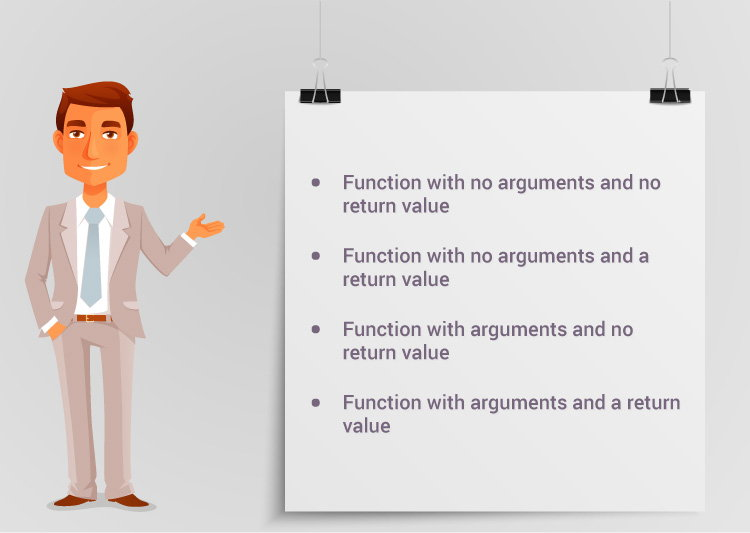# Types of User-defined Functions in C++

In this tutorial, you will learn about different approaches you can take to solve a single problem using functions.For better understanding of arguments and return in functions, user-defined functions can be categorised as:

Consider a situation in which you have to check prime number. This problem is solved below by making user-defined function in 4 different ways as mentioned above.

## Example 1: No arguments passed and no return value

``````# include <iostream>
using namespace std;

void prime();

int main()
{
// No argument is passed to prime()
prime();
return 0;
}

// Return type of function is void because value is not returned.
void prime()
{

int num, i, flag = 0;

cout << "Enter a positive integer enter to check: ";
cin >> num;

for(i = 2; i <= num/2; ++i)
{
if(num % i == 0)
{
flag = 1;
break;
}
}

if (flag == 1)
{
cout << num << " is not a prime number.";
}
else
{
cout << num << " is a prime number.";
}
}
``````

In the above program, `prime()` is called from the main() with no arguments.

`prime()` takes the positive number from the user and checks whether the number is a prime number or not.

Since, return type of `prime()` is `void`, no value is returned from the function.

## Example 2: No arguments passed but a return value

``````#include <iostream>
using namespace std;

int prime();

int main()
{
int num, i, flag = 0;

// No argument is passed to prime()
num = prime();
for (i = 2; i <= num/2; ++i)
{
if (num%i == 0)
{
flag = 1;
break;
}
}

if (flag == 1)
{
cout<<num<<" is not a prime number.";
}
else
{
cout<<num<<" is a prime number.";
}
return 0;
}

// Return type of function is int
int prime()
{
int n;

printf("Enter a positive integer to check: ");
cin >> n;

return n;
}``````

In the above program, `prime()` function is called from the `main()` with no arguments.

`prime()` takes a positive integer from the user. Since, return type of the function is an `int`, it returns the inputted number from the user back to the calling `main()` function.

Then, whether the number is prime or not is checked in the main() itself and printed onto the screen.

## Example 3: Arguments passed but no return value

``````#include <iostream>
using namespace std;

void prime(int n);

int main()
{
int num;
cout << "Enter a positive integer to check: ";
cin >> num;

// Argument num is passed to the function prime()
prime(num);
return 0;
}

// There is no return value to calling function. Hence, return type of function is void. */
void prime(int n)
{
int i, flag = 0;
for (i = 2; i <= n/2; ++i)
{
if (n%i == 0)
{
flag = 1;
break;
}
}

if (flag == 1)
{
cout << n << " is not a prime number.";
}
else {
cout << n << " is a prime number.";
}
}``````

In the above program, positive number is first asked from the user which is stored in the variable num.

Then, num is passed to the `prime()` function where, whether the number is prime or not is checked and printed.

Since, the return type of `prime()` is a `void`, no value is returned from the function.

## Example 4: Arguments passed and a return value.

``````#include <iostream>
using namespace std;

int prime(int n);

int main()
{
int num, flag = 0;
cout << "Enter positive integer to check: ";
cin >> num;

// Argument num is passed to check() function
flag = prime(num);

if(flag == 1)
cout << num << " is not a prime number.";
else
cout<< num << " is a prime number.";
return 0;
}

/* This function returns integer value.  */
int prime(int n)
{
int i;
for(i = 2; i <= n/2; ++i)
{
if(n % i == 0)
return 1;
}

return 0;
}
``````

In the above program, a positive integer is asked from the user and stored in the variable `num`.

Then, `num` is passed to the function `prime()` where, whether the number is prime or not is checked.

Since, the return type of `prime()` is an `int`, 1 or 0 is returned to the `main()` calling function. If the number is a prime number, 1 is returned. If not, 0 is returned.

Back in the `main()` function, the returned 1 or 0 is stored in the variable flag, and the corresponding text is printed onto the screen.

## Which method is better?

All four programs above gives the same output and all are technically correct program.

There is no hard and fast rule on which method should be chosen.

The particular method is chosen depending upon the situation and how you want to solve a problem.